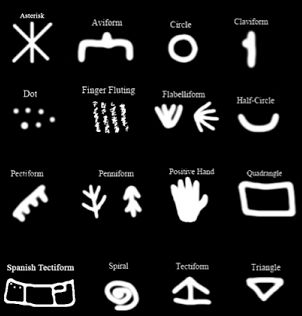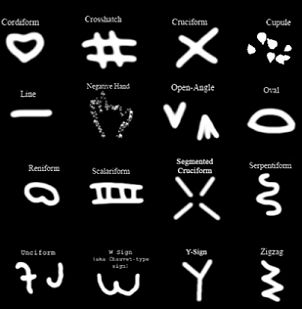Search IntMath
Close

# Geometric shapes in ancient rock art paintings

By Murray Bourne, 19 Apr 2016

In the video below, anthropologist Genevieve von Petzinger explains how certain symbols occur regularly in ancient rock art. Some of those cave markings are quite suggestive of mathematical symbols. This could be totally by accident, of course, but it's interesting to speculate beyond the main theme of her talk (that some aspects of written communication may be older than origninally thought) and consider the early development of mathematical and geometric symbols.

## Some examples from the talk

Here are some screen shots from the video, showing:

• A circle,
• Numeral "1"
• Half-circle,
• Spiral,
• TriangleIn this second image, we have what looks like rudimentary developmental symbols for:

• Hash,
• Multiply sign
• LineOf course, such symbols are only "mathematical" if they were used in a mathematical way, but when you consider many of our current mathematical symbols were just borrowed from existing languages (many of the commonly used Greek letters, for example), it is possible that in the ancient world, symbols had a double meaning as well.

On with the video, entitled Why Are These 32 Symbols Found in Caves All Over Europe:

The related Guardian article, Did Stone Age cavemen talk to each other in symbols? mentions an intriguing commonly occurring pattern in cave art and jewellery: II ^ III X II.

Perhaps this suggests rudimentary background symbols for the Roman numeral system, or perhaps they just found them "pretty". We tend to see what we want to see in unknown symbols.

### Comment Preview

HTML: You can use simple tags like <b>, <a href="...">, etc.

To enter math, you can can either:

1. Use simple calculator-like input in the following format (surround your math in backticks, or qq on tablet or phone):
a^2 = sqrt(b^2 + c^2)
(See more on ASCIIMath syntax); or
2. Use simple LaTeX in the following format. Surround your math with $$ and $$.
$$\int g dx = \sqrt{\frac{a}{b}}$$
(This is standard simple LaTeX.)

NOTE: You can mix both types of math entry in your comment.